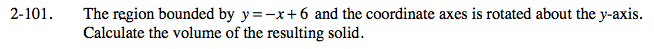### Home > CALC > Chapter 2 > Lesson 2.2.4 > Problem2-101

2-101.

The region bounded by y = − x + 6 and the coordinate axes is rotated about the y-axis. Calculate the volume of the resulting solid. Homework Help ✎Sketch the region so you can visualize the flag.

The axis of rotation (the pole) is the y-axis.

The resulting solid will be a cone.

Which axis contains the radius (r) and which contains the height (h)?